9TH MAHARASHTRA: MATHS I 10TH TAMIL NADU: MATHS, SCIENCE, SSLC 10TH NEW TEXT BOOK PDF DOWNLOAD CBSE 10TH: MATHS 2019 BOARD PAPER SOLUTION: 2019 BOARD PAPER SOLUTION

Continuity Ex. no. 3.1 Sum No. 2 Part Three

vii. f(x) = x^2 cos(1/x) [video]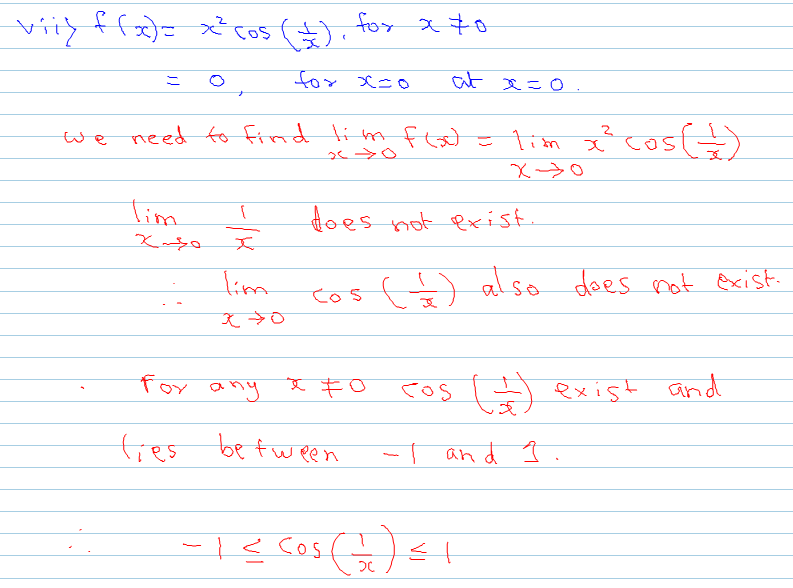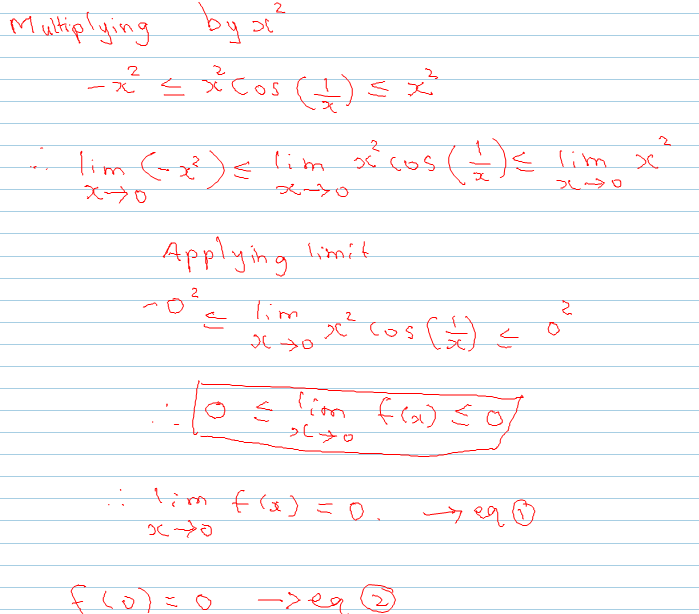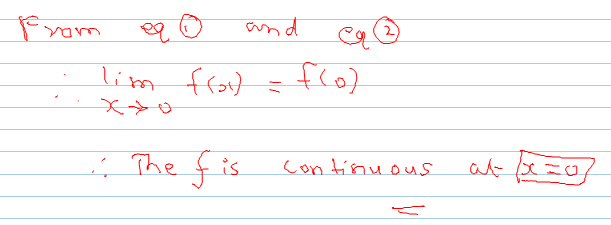viii. f(x) = (tanx - sinx) / (sin3x - 3sinx) [video]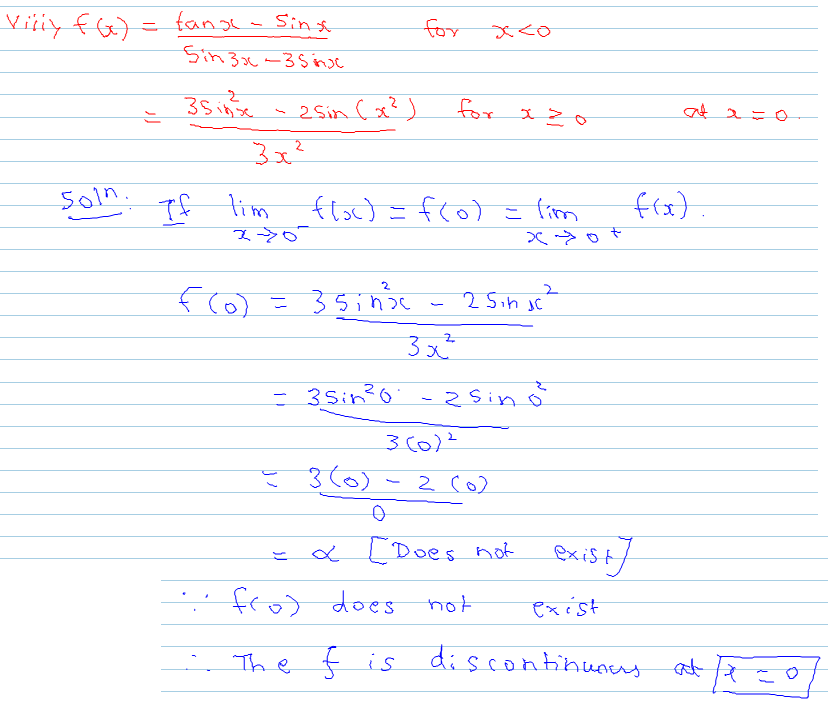ix. f(x) = x^3 + x^ 2 - 9x - 2 [video]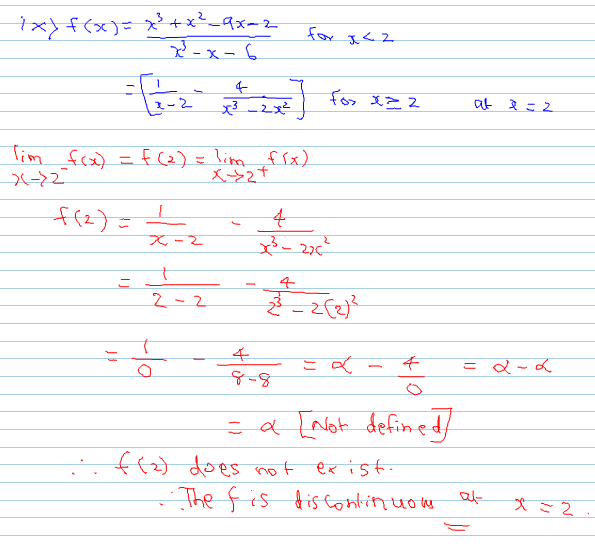x. f(x) = 6^x + 3^x - 2^x - 1 [video]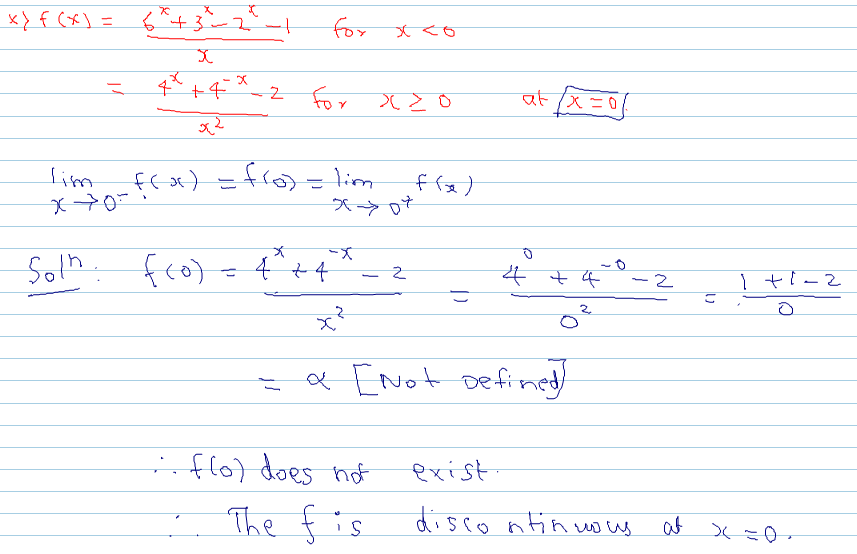Continuity Ex. No. 3.1 Sum No. 2 Part two

Continuity Ex. no. 3.1 Sum No. 2 Part Three